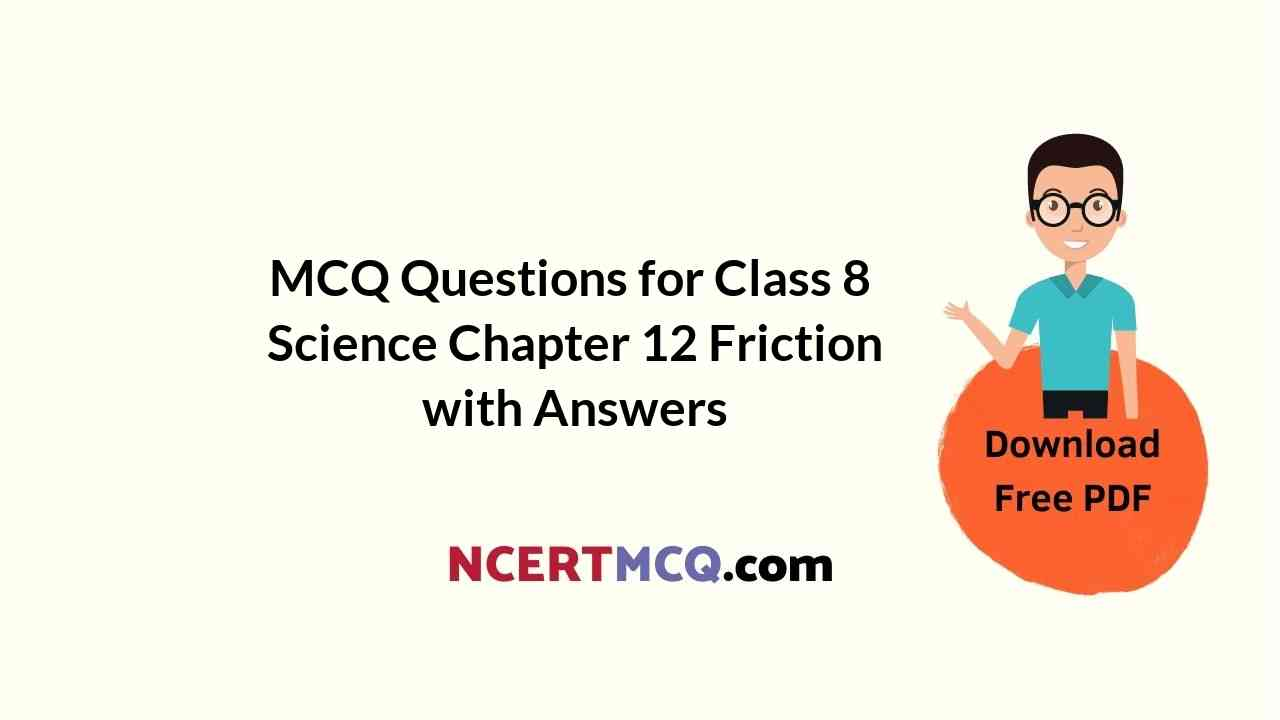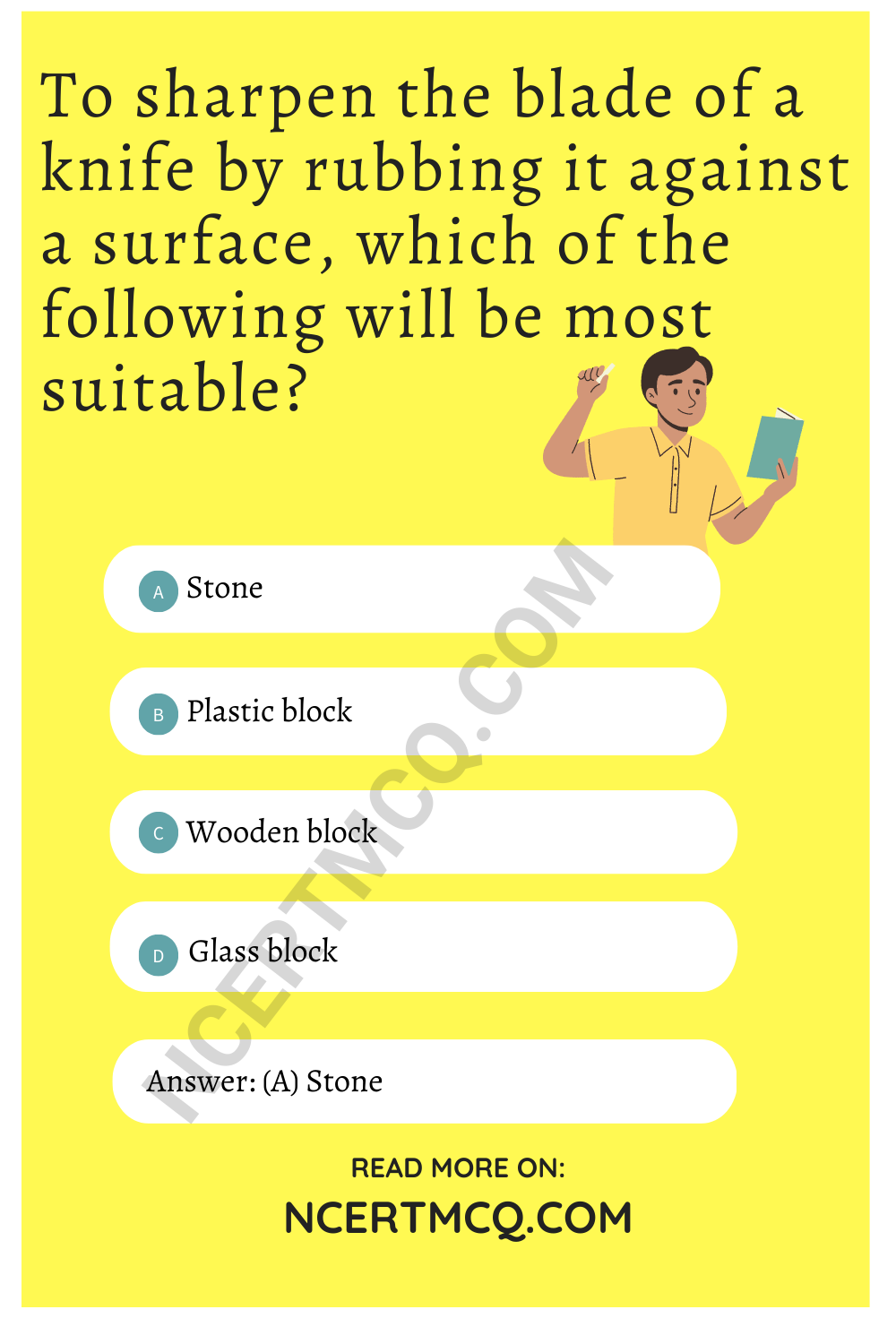Check the below Online Education NCERT MCQ Questions for Class 8 Science Chapter 12 Friction with Answers Pdf free download. MCQ Questions for Class 8 Science with Answers were prepared based on the latest exam pattern. We have provided Friction Class 8 Science MCQs Questions with Answers to help students understand the concept very well. https://ncertmcq.com/mcq-questions-for-class-8-science-with-answers/

You can refer to NCERT Solutions for Class 8 Science Chapter 12 Friction to revise the concepts in the syllabus effectively and improve your chances of securing high marks in your board exams.

## Online Education for Friction Class 8 MCQs Questions with Answers

Choose the correct option.

Friction Class 8 MCQ Question 1.
Friction is a
(a) non-contact force
(b) contact force
(c) magnetic force
(d) electrostatic force

Class 8 Science Chapter 12 MCQ Question 2.
Which of the following produces least friction?
(a) Sliding friction
(b) Rolling friction
(c) Composite friction
(d) Static friction

Friction MCQ Class 8 Question 3.
Friction always
(a) opposes the motion
(b) helps the motion
(c) both (a) and (b)
(d) none of these

Class 8 Friction MCQ Question 4.
Friction can be reduced by using
(a) oil
(b) grease
(c) powder
(d) all of these

MCQ On Friction Class 8 Question 5.
Static friction is less than
(a) sliding friction
(b) rolling friction
(c) both (a) and (b)
(d) none of these

MCQ Of Friction Class 8 Question 6.
Whenever the surfaces in contact tend to move or move with respect to each other, the force of friction comes into play
(a) only if the objects are solid.
(b) only if one of the two objects is liquid.
(c) only if one of the two objects is gaseous.
(d) irrespective of whether the objects are solid, liquid or gaseous.

Answer: (d) irrespective of whether the objects are solid, liquid or gaseous.

MCQ Questions For Class 8 Science Chapter 12 Question 7.
To sharpen the blade of a knife by rubbing it against a surface, which of the following will be most suitable?
(a) Stone
(b) Plastic block
(c) Wooden block
(d) Glass blockClass 8 Science Ch 12 MCQ Question 8.
The shape of an aeroplane is like a
(a) dog
(b) bird
(c) car
(d) all of these

Class 8 Science Friction MCQ Question 9.
Friction is
(a) foe
(b) friend
(c) both (a) and (b)
(d) none of these

Answer: (c) both (a) and (b)

Force And Friction Class 8 MCQ Question 10.
Friction due to fluids is called
(a) force
(b) pressure
(c) friction
(d) drag

MCQ Friction Class 8 Question 11.
Which of the following is not a smooth surface?
(a) Surface of wet soap
(b) Surface of tyres
(c) Glazed tiles
(d) Surface of mirror

Ch 12 Science Class 8 MCQ Question 12.
A toy car released with the same initial speed will travel farthest on
(a) muddy surface
(b) polished marble surface
(c) cemented surface
(d) brick surface

Class 8 Science Chapter Friction MCQ Question 13.
If we apply oil on door hinges, the friction will
(a) increase
(b) decrease
(c) disappear altogether
(d) will remain unchanged

Class 8 Chapter 12 Science MCQ Question 14.
Lubricant
(a) reduce friction
(b) increase friction
(c) both (a) and (b)
(d) none of these

MCQs On Friction Class 8 Question 15.
Force of friction depends on
(a) roughness of surface
(b) smoothness of surface
(c) inclination of surface
(d) all of these

Class 8 Ch 12 Science MCQ Question 16.
Force of friction depends on
(a) roughness of surface
(b) weight
(c) size
(d) all of these

Chapter 12 Science Class 8 MCQ Question 17.
Fluids are
(a) liquids
(b) gases
(c) both (a) and (b)
(d) none of these

Answer: (c) both (a) and (b)

Question 18.
Which is a streamlined object?
(a) Boats
(b) Aeroplanes
(c) Ships
(d) All of these

Question 19.
Smooth surface has
(a) less friction
(b) more friction
(c) sometimes less and sometimes more
(d) all of these

Question 20.
Which of the following is responsible for wearing out of bicycle tyres?
(a) Muscular force
(b) Magnetic force
(c) Frictional force
(d) Electrostatic force

Question 21.
It is difficult to walk on an oily floor because
(a) Floor gets spoiled
(b) There is more resistance
(c) Force of friction is high
(d) Force of friction is very less

Answer: (d) Force of friction is very less

Question 22.
Spring balance is a device used for measuring the _____________ acting on an object.
(a) mass
(b) pressure
(c) force
(d) None of the above

Question 23.
A matchstick struck on a matchbox catches fire easily because
(a) Friction may cause fire
(b) Of chemical reaction
(c) Force heated the match stick
(d) None of the above

Answer: (a) Friction may cause fire

Question 24.
(a) look good
(b) Increase friction
(c) increase its longivity
(d) increase weight of the tyre

Question 25.
Lubricants are substances which
(a) Increase friction
(b) Are used to light fire
(c) Reduce friction
(d) Are used to put out a fire

Question 26.
Sliding friction is _________ than / to rolling friction
(a) smaller
(b) greater
(c) equal
(d) None of the above

Question 27.
The frictional force exerted by fluids is also called ______
(a) drug
(b) drag
(c) drop
(d) drown

Question 28.
Four children were asked to arrange forces due to rolling, static and sliding frictions in an increasing order. Their arrangements are given below. Choose the correct arrangement.
(a) Rolling, Static, Sliding
(b) Static, Rolling, Sliding
(c) Rolling, sliding, static
(d) Sliding, Static, Rolling

Question 29.
A boat or an aeroplane has a pointed or tapering front / head. Why?
(a) To increase the friction of fluid
(b) To reduce the friction of fluid
(c) To look good
(d) For no reason

Answer: (b) To reduce the friction of fluid

Question 30.
The sole of the shoes becomes plain after wearing it for several months. The reason is
(a) Wearing out due to friction
(b) Wearing out due to no friction
(c) Sole is of bad quality
(d) None of the above

Answer: (a) Wearing out due to frictionMatch the items given in column I suitably with those given in column II.

 Column I Column II 1. Fluid friction (a) Due to friction 2. Lubricants (b) Streamlined 3. Wheels (c) Increases friction 4. Spring balance (d) Drag 5. Shape of aeroplane (e) Rolling friction 6. Rough surface (f) Reduce friction 7. Heat generation (g) Measures force

 Column I Column II 1. Fluid friction (d) Drag 2. Lubricants (f) Reduce friction 3. Wheels (e) Rolling friction 4. Spring balance (g) Measures force 5. Shape of aeroplane (b) Streamlined 6. Rough surface (c) Increases friction 7. Heat generation (a) Due to friction

Fill in the blanks with suitable word/s.

1. Sliding friction is ___________ than static friction.

2. Friction can be reduced by using ___________.

Answer: oil or powder or grease

3. Friction can be increased by making surface ___________.

4. Rolling friction is ___________ than sliding and static friction.

5. Friction due to fluid is also called ___________.

6. Bodies of birds, fishes and ships are ___________.

7. Friction can also produce ___________.

8. All objects moving in fluids have ___________ shape to reduce ___________.

9. Rough surfaces produce ___________ friction than smooth surfaces.

10. In many machines, friction is reduced by using ___________.

11. Friction depends on the ___________ of substances in contact.

12. Static friction comes into play when we try to move an object at ___________.

13. ___________ force is responsible for downward movement of a parachutist when he jumps from an aircraft

14. The sole of shoes and the tyres of vehicles are treaded to _________ friction.

15. Friction is sometimes _________ .

State whether the given statements are true or false.

1. Friction is always useful to us.

2. Fluids are only liquids.

3. Friction is less for smooth surfaces.

4. A soapy floor is slippery due to increased friction.

5. Friction always works in opposite direction to the relative motion.

6. Friction is dependent on area of contact.

7. Sliding friction is less than rolling friction.

8. Friction decreases with increase in the smoothness of the surface.

9. It is easier to move a heavy object than a light object.

10. Friction can be reduced by using lubricants.

11. Friction is independent of the nature of surfaces in contact.

12. A spring balance measures force.

13. Friction can be reduced by using ball bearings in some machines.

14. Friction can never be eliminated.